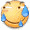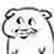# ouuan 的博客# ouuan 的博客

### 一些好用的网站

posted on 2018-07-19 15:37:20 | under 收藏 |

$\text{图床}$

$\text{几何}$

$\text{图论}$

$\text{函数}$

$\text{找规律}$

$\text{矩阵运算}$

$\text{在线汇编编译器}$

$\mathrm{C++}$

$\LaTeX\text{公式大全}$

$\LaTeX\text{公式奆全}$

$\text{.tex}$ 教程

$\text{OI Wiki}$

$\color{red}\mathrm{Wolfram}\color{orange}\mathrm{Alpha}$

$\color{white}\colorbox{black}{oier数据库}$![](https://i.loli.net/2018/10/23/5bcead9b66b11.gif)![](https://i.loli.net/2018/10/15/5bc3d11b9bd87.png)![](https://i.loli.net/2018/10/27/5bd4705dd844f.jpg)
#pragma GCC diagnostic error "-std=c++11"
#pragma GCC target("avx","sse2")